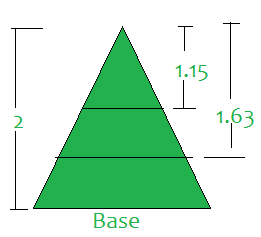# Split given isosceles triangle of height H into N equal parts

• Difficulty Level : Easy
• Last Updated : 18 Jun, 2021

Given an integer N and an isosceles triangle consisting of height H, the task is to find (N – 1) points on the triangle such that the line passing through these points and parallel to the base of the triangle, divide the total area into N equal parts.

Examples:

Attention reader! Don’t stop learning now. Get hold of all the important mathematical concepts for competitive programming with the Essential Maths for CP Course at a student-friendly price. To complete your preparation from learning a language to DS Algo and many more,  please refer Complete Interview Preparation Course.

Input: N = 3, H = 2
Output: 1.15 1.63
Explanation: Make cuts at point 1.15 and 1.63 as shown below:Input: N = 2, H = 1000
Output: 70710.67

Approach: The problem can be solved by observing the following properties:

Divide the triangle such that (xi / h)2 = i / N
=> xi = h*√(i/n)
xi = height of ith cut from the top vertex of the triangle

Follow the steps below to solve the problem:

1. Iterate over the range [1, N – 1].
2. In every ith iteration, print the value of xi using the above formula.

Below is the implementation of the above approach:

## C++

 `// C++ Code for above approach``#include ``using` `namespace` `std;` `// Function to divide the isosceles triangle``// in equal parts by making N-1 cuts``// parallel to the base``void` `findPoint(``int` `n, ``int` `h)``{``  ` `    ``// Iterate over the range [1, n - 1]``    ``for` `(``int` `i = 1; i < n; i++)``        ``printf``(``"%.2f "``, ``sqrt``(i / (n*1.0)) * h);``}` `// Driver code``int` `main()``{``  ``// Given N``  ``int` `n = 3;` `  ``// Given H``  ``int` `h = 2;` `  ``// Function call``  ``findPoint(n, h);` `  ``return` `0;``}` `// This code is contributed by mohit kumar 29`

## Java

 `// Java Code for above approach``import` `java.util.*;``class` `GFG``{` `    ``// Function to divide the isosceles triangle``    ``// in equal parts by making N-1 cuts``    ``// parallel to the base``    ``static` `void` `findPoint(``int` `n, ``int` `h)``    ``{` `        ``// Iterate over the range [1, n - 1]``        ``for` `(``int` `i = ``1``; i < n; i++)``            ``System.out.printf(``"%.2f "``,``                    ``Math.sqrt(i / (n * ``1.0``)) * h);``    ``}` `    ``// Driver code``    ``public` `static` `void` `main(String[] args)``    ``{``      ` `        ``// Given N``        ``int` `n = ``3``;` `        ``// Given H``        ``int` `h = ``2``;` `        ``// Function call``        ``findPoint(n, h);``    ``}``}` `// This code is contributed by shikhasingrajput`

## Python3

 `# Python Code for above approach`  `# Function to divide the isosceles triangle``# in equal parts by making N-1 cuts``# parallel to the base``def` `findPoint(n, h):`  `    ``# Iterate over the range [1, n - 1]``    ``for` `i ``in` `range``(``1``, n):``        ``print``(``"{0:.2f}"``.``format``(((i ``/` `n) ``*``*` `0.5``) ``*` `h), end ``=``' '``)`  `# Driver Code``if` `__name__ ``=``=` `'__main__'``:` `    ``# Given N``    ``n ``=` `3` `    ``# Given H``    ``h ``=` `2` `    ``# Function call``    ``findPoint(n, h)`

## C#

 `// C# Code for above approach``using` `System;``class` `GFG``{` `  ``// Function to divide the isosceles triangle``  ``// in equal parts by making N-1 cuts``  ``// parallel to the base``  ``static` `void` `findPoint(``int` `n, ``int` `h)``  ``{` `    ``// Iterate over the range [1, n - 1]``    ``for` `(``int` `i = 1; i < n; i++)``      ``Console.Write(``"{0:F2} "``,``                    ``Math.Sqrt(i / (n * 1.0)) * h);``  ``}` `  ``// Driver code``  ``public` `static` `void` `Main(String[] args)``  ``{` `    ``// Given N``    ``int` `n = 3;` `    ``// Given H``    ``int` `h = 2;` `    ``// Function call``    ``findPoint(n, h);``  ``}``}` `// This code is contributed by shikhasingrajput`

## Javascript

 `   `
Output:
`1.15 1.63`

Time Complexity: O(N)
Auxiliary Space: O(1)

My Personal Notes arrow_drop_up